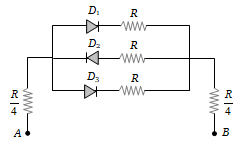In the following circuits PN-junction diodes ${\mathrm{D}}_{1}$${\mathrm{D}}_{2}$ and ${\mathrm{D}}_{3}$ are ideal for the following potential of A and B, the correct increasing order of resistance between A and B will be(i) – 10 V, – 5V           (ii) – 5V, – 10 V
(iii) – 4V, – 12V

(a) (i) < (ii) < (iii)                       (b) (iii) < (ii) < (i)
(c) (ii) = (iii) < (i)                       (d) (i) = (iii) < (ii)

Explanation is a part of a Paid Course. To view Explanation Please buy the course.

Difficulty Level: